# Asymptotic Matching

The resonant layer equations, (5.57)–(5.60), possess independent tearing parity solutions, characterized by the symmetry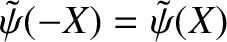,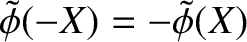,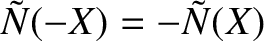,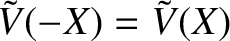, and twisting parity solutions, characterized by the symmetry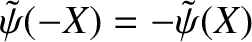,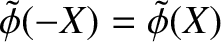,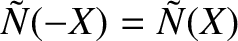,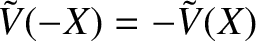. However, only the tearing parity solutions can be asymptotically matched to tearing mode solutions in the outer region. If we assume that the asymptotic behavior of the tearing parity layer solutions is such that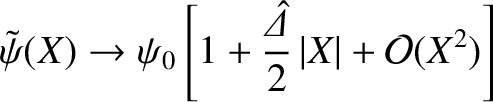(5.69)

as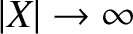, where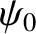is an arbitrary constant, then asymptotic matching to the outer solution yields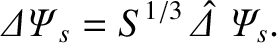(5.70)

[See Equations (3.72), (3.73), and (5.56).] Furthermore, it follows from Equations (3.19) and (3.58) that the asymptotic behavior of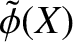is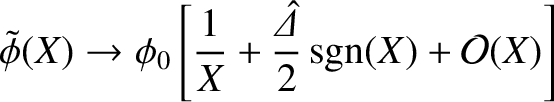(5.71)

as, where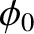is an arbitrary constant,